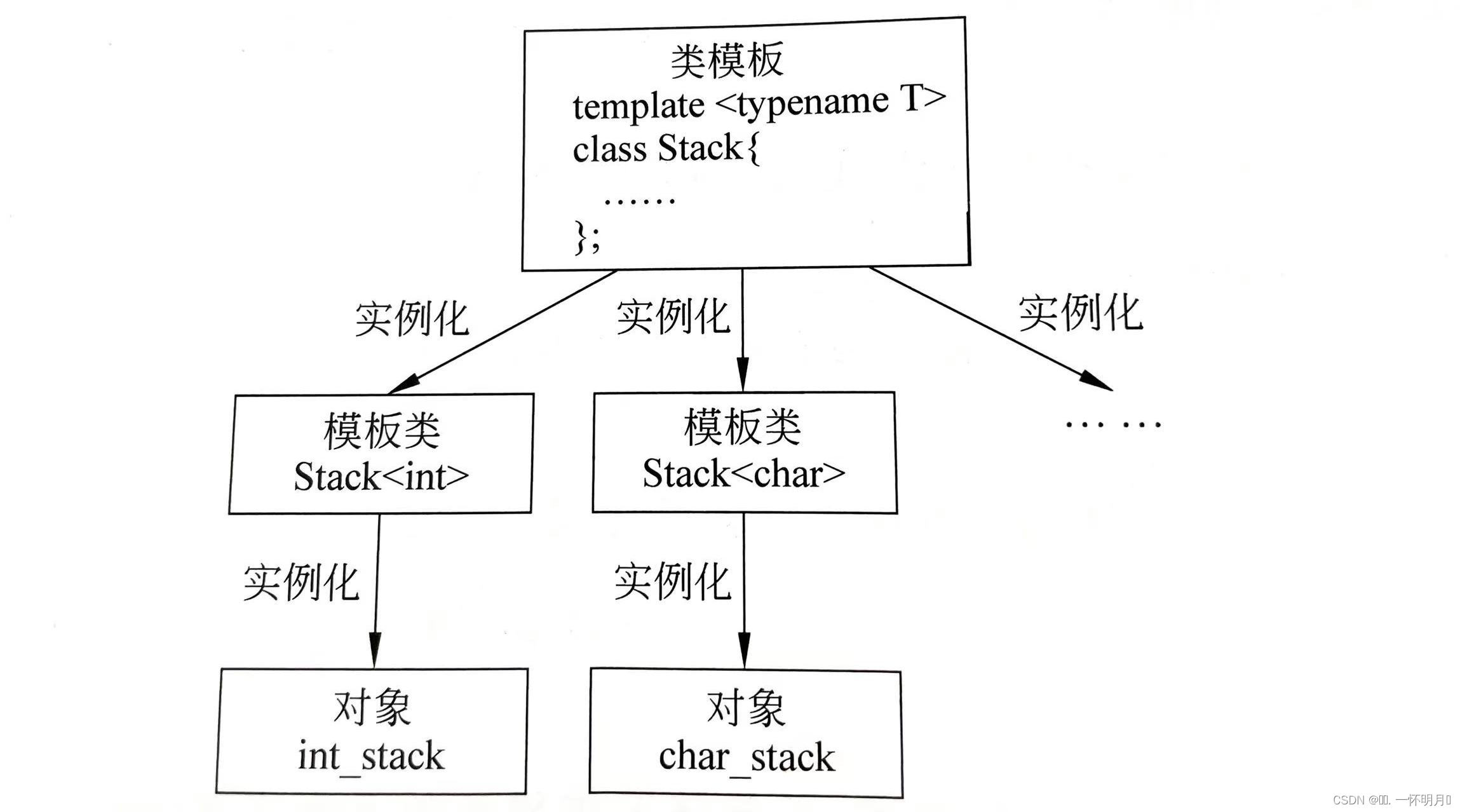# C++中的类模版

🐶博主主页：@ᰔᩚ. 一怀明月ꦿ

❤️‍🔥专栏系列：线性代数，C初学者入门训练，题解C，C的使用文章，「初学」C++

🔥座右铭：“不要等到什么都没有了，才下定决心去做”

🚀🚀🚀大家觉不错的话，就恳求大家点点关注，点点小爱心，指点指点🚀🚀🚀

🐰类模版

🌸类模版的声明

🌸类模版的实例化

🌸类模版参数

🌸默认模版实参

# 🐰类模版

## 🌸类模版的声明

template<typename T1,typename T2,...>
class 类名
{
类体
};

#include<iostream>
using namespace std;
const int SSize=10;//SSize为栈的容量
template<typename T>
class Stack
{
public:
Stack()
{
top=0;
}
void push(T e);//入栈
T pop();//出栈
bool stackEmpty()//判断栈是否为空
{
return top==0;
}
bool stackFull()//判断栈是否为满
{
return top==SSize;
}
private:
T data[SSize];
int top;
};
template<typename T>
void Stack<T>::push(T e)
{
if(stackFull())
{
cout<<"Stack is Full! Don't push data!"<<endl;
return ;
}
data[top]=e;
top++;
}
template<typename T>
T Stack<T>::pop()
{
if(stackEmpty())
{
cout<<"Stack is Empty! Don't pop data!"<<endl;
return ;
}
top--;
return data[top];
}

（1）类模版中的成员函数即可以在类模版内定义，也可以在类模版外定义

template<模版参数列表>

{
函数体
}

Stack中的push成员函数

template<typename T>
void Stack<T>::push(T e)
{
if(stackFull())
{
cout<<"Stack is Full! Don't push data!"<<endl;
return ;
}
data[top]=e;
top++;
}

（2）如果在类模版外将成员函数定义为inline（内联）函数，应将inline关键字加在类模版的声明后。

template<typename T>
inline T Stack<T>::pop(){...}

（3）类模版的成员函数的定义必须同类模版的定义在同一个文件中因为，类模版定义不同与类的定义，编译器无法通过一般的手段找到类模版的成员函数的代码，只有将它和类模版定义放一起，才能保证类模版正常使用。一般都放在一个头文件中（.h文件中）

## 🌸类模版的实例化

Stack<int>int_stack;

class Stack
{
public:
Stack()
{
top=0;
}
void push(int e);//入栈
int pop();//出栈
bool stackEmpty()//判断栈是否为空
{
return top==0;
}
bool stackFull()//判断栈是否为满
{
return top==SSize;
}
private:
int data[SSize];
int top;
};类模版名<实际模版参数列表>对象名;

## 🌸类模版参数

#include<iostream>
using namespace std;
template<typename T,int SSize>
class Stack
{
public:
Stack()
{
top=0;
}
void push(T e);//入栈
T pop();//出栈
bool stackEmpty()//判断栈是否为空
{
return top==0;
}
bool stackFull()//判断栈是否为满
{
return top==SSize;
}
private:
T data[SSize];
int top;
};
template<typename T,int SSize>
void Stack<T,SSize>::push(T e)
{
if(stackFull())
{
cout<<"Stack is Full! Don't push data!"<<endl;
return ;
}
data[top]=e;
top++;
}
template<typename T,int SSize>
T Stack<T,SSize>::pop()
{
if(stackEmpty())
{
cout<<"Stack is Empty! Don't pop data!"<<endl;
return ;
}
top--;
return data[top];
}

Stack<int,10>int_stack;

## 🌸默认模版实参

template<typename T,int SSize=10>
class Stack
{
public:
Stack()
{
top=0;
}
void push(T e);//入栈
T pop();//出栈
bool stackEmpty()//判断栈是否为空
{
return top==0;
}
bool stackFull()//判断栈是否为满
{
return top==SSize;
}
private:
T data[SSize];
int top;
};

（1）作为默认的模版实参，它们只能被定义一次，编译器会知道第一次的模版声明或定义

（2）指定默认实参的模版参数必须放在模版形参列表的右端，否则出错（和重载函数参数列表一样，带有默认值的参数必须放右端）

template<typename T1,typename T2,typename T3=int,int SSize=10>//正确
template<typename T1,typename T2=int,typename T3,int SSize=10>//错误

（3）可以为所有的模版参数提供实参但声明，如果用Stack类模版实例化一个对象时，如果全部都想使用模版参数的默认值，就必须使用一对尖括号，这样编译器就知道说明了一个类模版例如：

#include<iostream>
using namespace std;
template<typename T=int,int SSize=10>
class Stack
{
public:
Stack()
{
top=0;
}
void push(T e);//入栈
T pop();//出栈
bool stackEmpty()//判断栈是否为空
{
return top==0;
}
bool stackFull()//判断栈是否为满
{
return top==SSize;
}
private:
T data[SSize];
int top;
};
template<typename T,int SSize>//这里千万不要写成template<typename T=int,int SSize=10>，因为作为默认的模版实参，它们只能被定义一次
void Stack<T,SSize>::push(T e)
{
if(stackFull())
{
cout<<"Stack is Full! Don't push data!"<<endl;
return ;
}
data[top]=e;
top++;
}
template<typename T,int SSize>//这里千万不要写成template<typename T=int,int SSize=10>，因为作为默认的模版实参，它们只能被定义一次
T Stack<T,SSize>::pop()
{
if(stackEmpty())
{
cout<<"Stack is Empty! Don't pop data!"<<endl;
return 1;
}
top--;
return data[top];
}
int main()
{
Stack<>int_stack;//注意空的尖括号，这里全部是用类模版参数的默认值
int_stack.push(5);
cout<<int_stack.pop()<<endl;
return 0;
}

5


🌸🌸🌸如果大家还有不懂或者建议都可以发在评论区，我们共同探讨，共同学习，共同进步。谢谢大家！ 🌸🌸🌸